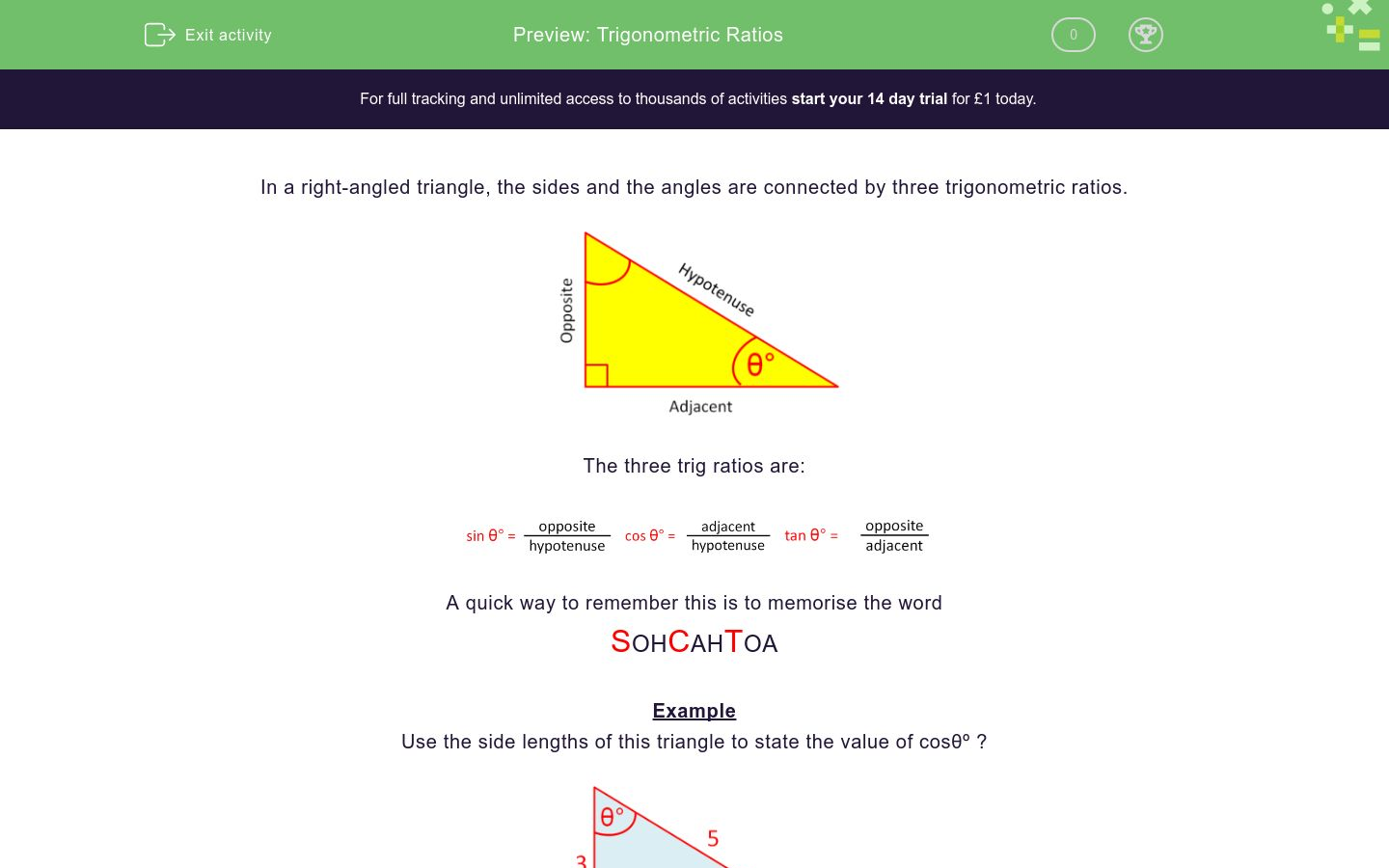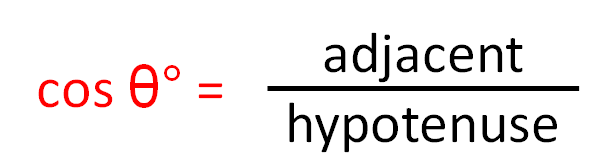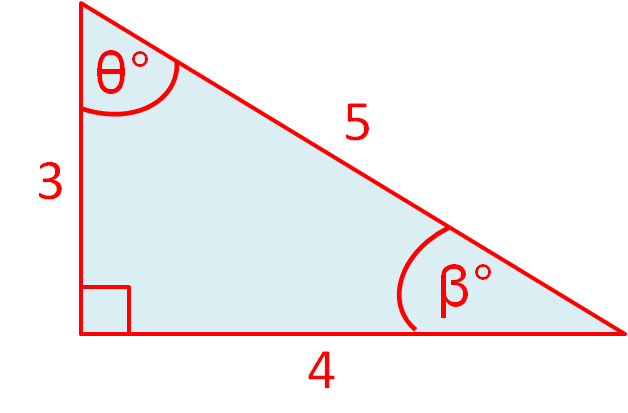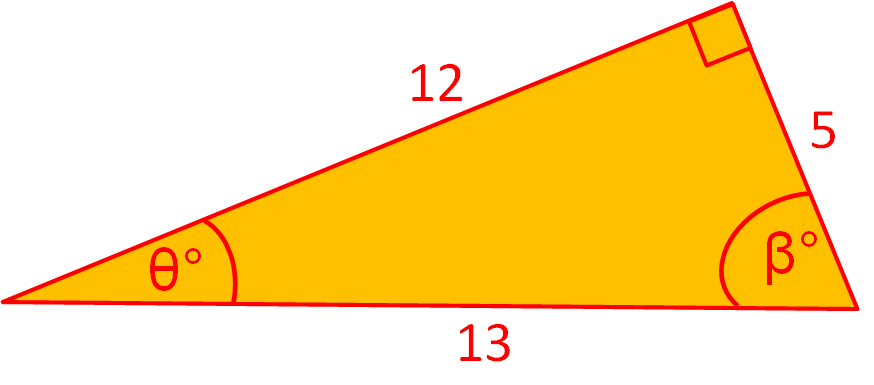# Trigonometric Ratios

In this worksheet, student work out the given trigonometric ratio in right-angled triangles.Key stage:  KS 3

Curriculum topic:   Geometry and Measures

Curriculum subtopic:   Solve Right-Angled Triangle Problems

Difficulty level:### QUESTION 1 of 10

In a right-angled triangle, the sides and the angles are connected by three trigonometric ratios.The three trig ratios are:A quick way to remember this is to memorise the word

SOHCAHTOA

Example

Use the side lengths of this triangle to state the value of cosθº ?Label the sides adjacent, opposite and hypotenuse in relation to the angle θº.SOHCAHTOA

The side adjacent to the angle θ has length 3.

The hypotenuse has length 5.

The correct trig ratio is COS so cos θº= 3/5

Use the side lengths of this triangle to state the value of sinθº ?3/5

4/5

3/4

5/3

5/4

4/3

Use the side lengths of this triangle to state the value of tanθº ?3/5

4/5

3/4

5/3

5/4

4/3

Use the side lengths of this triangle to state the value of tanβº ?3/5

4/5

3/4

5/3

5/4

4/3

Use the side lengths of this triangle to state the value of sinβº ?3/5

4/5

3/4

5/3

5/4

4/3

Use the side lengths of this triangle to state the value of cosβº ?3/5

4/5

3/4

5/3

5/4

4/3

Use the side lengths of this triangle to state the value of sinθº ?5/12

5/13

12/13

13/12

13/5

12/5

Use the side lengths of this triangle to state the value of cosθº ?5/12

5/13

12/13

13/12

13/5

12/5

Use the side lengths of this triangle to state the value of tanθº ?5/12

5/13

12/13

13/12

13/5

12/5

Use the side lengths of this triangle to state the value of cosβº ?5/12

5/13

12/13

13/12

13/5

12/5

Use the side lengths of this triangle to state the value of tanβº ?5/12

5/13

12/13

13/12

13/5

12/5

• Question 1

Use the side lengths of this triangle to state the value of sinθº ?4/5
• Question 2

Use the side lengths of this triangle to state the value of tanθº ?4/3
• Question 3

Use the side lengths of this triangle to state the value of tanβº ?3/4
• Question 4

Use the side lengths of this triangle to state the value of sinβº ?3/5
• Question 5

Use the side lengths of this triangle to state the value of cosβº ?4/5
• Question 6

Use the side lengths of this triangle to state the value of sinθº ?5/13
• Question 7

Use the side lengths of this triangle to state the value of cosθº ?12/13
• Question 8

Use the side lengths of this triangle to state the value of tanθº ?5/12
• Question 9

Use the side lengths of this triangle to state the value of cosβº ?5/13
• Question 10

Use the side lengths of this triangle to state the value of tanβº ?12/5
---- OR ----

Sign up for a £1 trial so you can track and measure your child's progress on this activity.

### What is EdPlace?

We're your National Curriculum aligned online education content provider helping each child succeed in English, maths and science from year 1 to GCSE. With an EdPlace account you’ll be able to track and measure progress, helping each child achieve their best. We build confidence and attainment by personalising each child’s learning at a level that suits them.

Get started